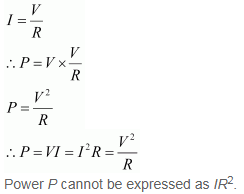3 Tutor System
Starting just at 265/hour

# Which of the following terms does not represent electrical power in a circuit? (a)$$I^2R$$ (b) $$IR^2$$ (c)VI (d) $$V^2$$/R

(b)$$IR^2$$